•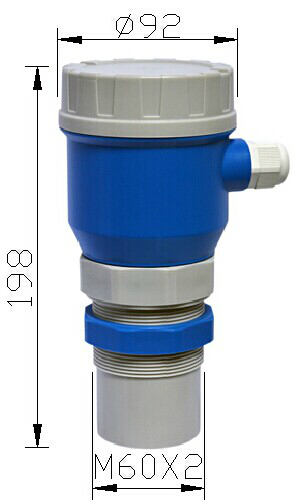防腐防爆高频微 `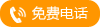` `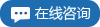`
•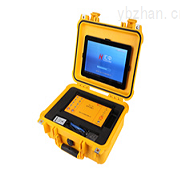便携式超声波流 `` ``
•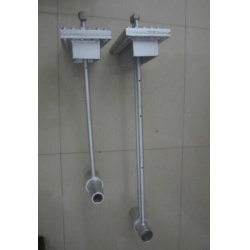YY-DHL单点多喉 `` ``
•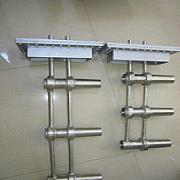多点多喉径流量 `` ``
•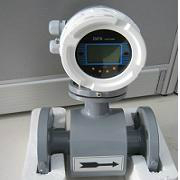高精度电磁流量 `` ``
•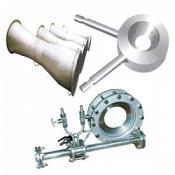流量测量节流装 `` ``
•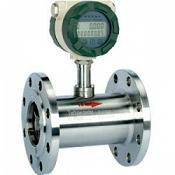液体涡轮流量计 `` ``
•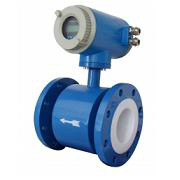一体式电磁流量 `` ``
•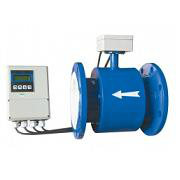污水流量计(分体 `` ``
•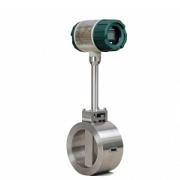智能一体式涡街 `` ``
•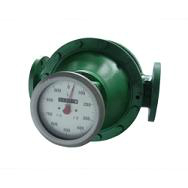椭圆齿轮流量计 `` ``
•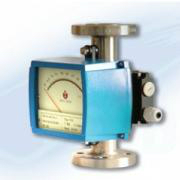金属管转子流量 `` ``
•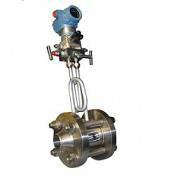一体化孔板流量 `` ``
•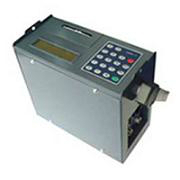便携式超声波流 `` ``
•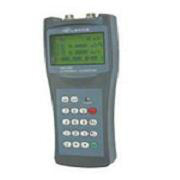手持式超声波流 `` ``
•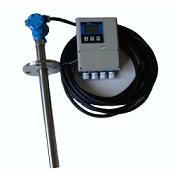插入式电磁流量 `` ``
•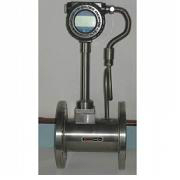带温压补偿涡街 `` ``
•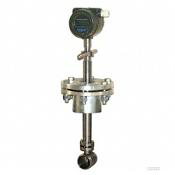插入式涡街流量 `` ``
•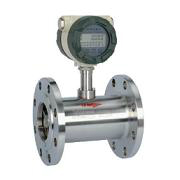气体涡轮流量计 `` ``
•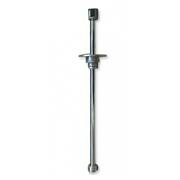插入式涡轮流量 `` ``